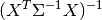# statsmodels.regression.linear_model.GLS¶

class `statsmodels.regression.linear_model.``GLS`(endog, exog, sigma=None, missing='none', hasconst=None, **kwargs)[source]

Generalized least squares model with a general covariance structure.

Parameters: endog : array-like 1-d endogenous response variable. The dependent variable. exog : array-like A nobs x k array where nobs is the number of observations and k is the number of regressors. An intercept is not included by default and should be added by the user. See `statsmodels.tools.add_constant`. sigma : scalar or array sigma is the weighting matrix of the covariance. The default is None for no scaling. If sigma is a scalar, it is assumed that sigma is an n x n diagonal matrix with the given scalar, sigma as the value of each diagonal element. If sigma is an n-length vector, then sigma is assumed to be a diagonal matrix with the given sigma on the diagonal. This should be the same as WLS. missing : str Available options are ‘none’, ‘drop’, and ‘raise’. If ‘none’, no nan checking is done. If ‘drop’, any observations with nans are dropped. If ‘raise’, an error is raised. Default is ‘none.’ hasconst : None or bool Indicates whether the RHS includes a user-supplied constant. If True, a constant is not checked for and k_constant is set to 1 and all result statistics are calculated as if a constant is present. If False, a constant is not checked for and k_constant is set to 0. **Attributes** pinv_wexog : array pinv_wexog is the p x n Moore-Penrose pseudoinverse of wexog. cholsimgainv : array The transpose of the Cholesky decomposition of the pseudoinverse. df_model : float p - 1, where p is the number of regressors including the intercept. of freedom. df_resid : float Number of observations n less the number of parameters p. llf : float The value of the likelihood function of the fitted model. nobs : float The number of observations n. normalized_cov_params : array p x p arrayresults : RegressionResults instance A property that returns the RegressionResults class if fit. sigma : array sigma is the n x n covariance structure of the error terms. wexog : array Design matrix whitened by cholsigmainv wendog : array Response variable whitened by cholsigmainv

Notes

If sigma is a function of the data making one of the regressors a constant, then the current postestimation statistics will not be correct.

Examples

```>>> import numpy as np
>>> import statsmodels.api as sm
>>> ols_resid = sm.OLS(data.endog, data.exog).fit().resid
>>> res_fit = sm.OLS(ols_resid[1:], ols_resid[:-1]).fit()
>>> rho = res_fit.params
```

rho is a consistent estimator of the correlation of the residuals from an OLS fit of the longley data. It is assumed that this is the true rho of the AR process data.

```>>> from scipy.linalg import toeplitz
>>> order = toeplitz(np.arange(16))
>>> sigma = rho**order
```

sigma is an n x n matrix of the autocorrelation structure of the data.

```>>> gls_model = sm.GLS(data.endog, data.exog, sigma=sigma)
>>> gls_results = gls_model.fit()
>>> print(gls_results.summary()))
```

Methods

 `fit`([method, cov_type, cov_kwds, use_t]) Full fit of the model. `fit_regularized`([method, maxiter, alpha, ...]) Return a regularized fit to a linear regression model. `initialize`() `loglike`(params) Returns the value of the Gaussian log-likelihood function at params. `predict`(params[, exog]) Return linear predicted values from a design matrix. `whiten`(X) GLS whiten method.

Attributes

 `df_model` The model degree of freedom, defined as the rank of the regressor matrix minus 1 if a constant is included. `df_resid` The residual degree of freedom, defined as the number of observations minus the rank of the regressor matrix. `endog_names` `exog_names`